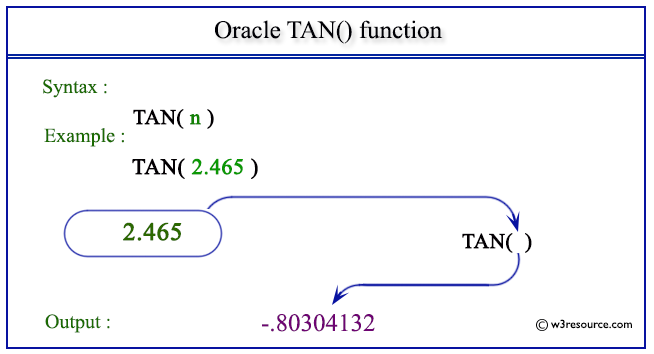# Oracle TAN() function

## Description

This function returns the tangent of n (an angle expressed in radians).
The function takes any numeric or nonnumeric data type (can be implicitly converted to a numeric data type) as an argument.
If the argument is BINARY_FLOAT, then the function returns BINARY_DOUBLE. Otherwise, the function returns the same numeric data type as the argument

Syntax:

`TAN(n)`

Where n is a number

Pictorial Presentation of TAN() functionExample - 1

``````SELECT TAN(2.465)FROM dual;
```
```

Here is the result.

```TAN(2.465)
----------
-.80304132
```

The above statement will return the tangent of the given number 2.465.

Example - 2

``````SELECT TAN(110 * 3.14159265359/180)
"Tangent of 110 degrees"  FROM DUAL;
```
```

Here is the result.

```Tangent of 110 degrees
----------------------
-2.7474774
```

The above statement will return the tangent of the given expression in radians.

Previous: SQRT
Next: TANH

﻿